# Linear and Quadratic Discriminant Analysis¶

Exploring the theory and implementation behind two well known generative classification algorithms: Linear discriminative analysis (LDA) and Quadratic discriminative analysis (QDA)

This notebook will use the Iris dataset as a case study for comparing and visualizing the prediction boundaries of the algorithms.

## Linear Discriminant Analysis (LDA)¶

For we assume that the random variable $X$ is a vector $\mathbf{X} = (X_1,X_2,...,X_p)$ which is drawn from a multivariate Gaussian with class-specific mean vector and a common covariance matrix $\Sigma$. In other words the covariance matrix is common to all $K$ classes: $Cov(X) = \Sigma$ of shape $p \times p$

Since $x$ follows a multivariate Gaussian distribution, the probability $p(X = x | Y = k)$ is given by: ($\mu_k$ is the mean of inputs for category $k$)

$$f_k(x) = \frac{1}{(2 \pi)^{p/2} |\Sigma|^{1/2}} \exp \left( - \frac{1}{2} (x - \mu_k)^T \Sigma^{-1} (x - \mu_k) \right)$$

Assume that we know the prior distribution exactly: $P(Y = k) = \pi_k$, then we can find the posterior distribution as

$$p(Y = k | X = x) = \frac{f_k(x) \ \pi_k}{P(X = x)} = C \times f_k(x) \ \pi_k$$

Since $P(X = x)$ does not depend on $k$ so we write it as a constant. We will now proceed to expand and simplify the algebra, putting all constant terms into $C, C', C''$ etc..

\begin{aligned} p_k(x) &= p(Y = k | X = x) = \frac{f_k(x) \ \pi_k}{P(X = x)} = C \times f_k(x) \ \pi_k \\ & = C \ \pi_k \ \frac{1}{(2 \pi)^{p/2} |\Sigma|^{1/2}} \exp \left( - \frac{1}{2} (x - \mu_k)^T \Sigma^{-1} (x - \mu_k) \right) \\ & = C' \pi_k \ \exp \left( - \frac{1}{2} (x - \mu_k)^T \Sigma^{-1} (x - \mu_k) \right) \end{aligned}

Take the log of both sides:

\begin{aligned} \log p_k(x) &= \log ( C' \pi_k \ \exp \left( - \frac{1}{2} (x - \mu_k)^T \Sigma^{-1} (x - \mu_k) \right) ) \\ & = \log C' + \log \pi_k - \frac{1}{2} (x - \mu_k)^T \Sigma^{-1} (x - \mu_k) \end{aligned}

Since the term $\log C'$ does not depend on $k$ and we aim to maximize the posterior probability over $k$, we can ignore it:

\begin{aligned} \log \pi_k - \frac{1}{2} (x - \mu_k)^T \Sigma^{-1} (x - \mu_k) \\ = \log \pi_k - \frac{1}{2} [ x^T \Sigma^{-1} x + \mu^T_k \Sigma^{-1} \mu_k ] + x^T \Sigma^{-1} \mu_k \\ = C'' + \log \pi_k - \frac{1}{2} \mu^T_k \Sigma^{-1} \mu_k + x^T \Sigma^{-1} \mu_k \end{aligned}

And so the objective function, sometimes called the linear discriminant function or linear score function is:

$$\delta_k(x) = \log \pi_k - \frac{1}{2} \mu^T_k \Sigma^{-1} \mu_k + x^T \Sigma^{-1} \mu_k$$

Which means that given an input $x$ we predict the class with the highest value of $\delta_k(x)$.

## Decision boundary¶

The next question is, what is the decision boundary ? It is the set of points in which two classes are equally probable:

$$\delta_k(x) = \delta_l(x)$$ $$\log \pi_k - \frac{1}{2} \mu^T_k \Sigma^{-1} \mu_k + x^T \Sigma^{-1} \mu_k = \log \pi_l - \frac{1}{2} \mu^T_l \Sigma^{-1} \mu_l + x^T \Sigma^{-1} \mu_l$$

or equivalently by equating to zero

$$\log \frac{\pi_k}{\pi_l} - \frac{1}{2} (\mu_k + \mu_l)^T \Sigma^{-1} (\mu_k - \mu_l) + x^T \Sigma^{-1} (\mu_k - \mu_l) = 0$$

Which is a linear function in $x$ - this explains why the decision boundaries are linear - hence the name Linear Discriminant Analysis.

### Example¶

As a simple worked example, assume we have found the following:

• $\pi_1 = \pi_2 = .5$
• $\mu_1 = (0,0)^T$ and $\mu_2 = (2,-2)^T$
• $\Sigma = \begin{bmatrix} 1.0 & 0.0 \\ 0.0 & 0.5625 \end{bmatrix}$

The decision boundary is given by

$$\log \frac{.5}{.5} - \frac{1}{2} (2,-2) \begin{bmatrix} 1.0 & 0.0 \\ 0.0 & 0.5625 \end{bmatrix} \begin{bmatrix} -2 \\ 2 \end{bmatrix} + (x_1, x_2) \begin{bmatrix} 1.0 & 0.0 \\ 0.0 & 0.5625 \end{bmatrix} \begin{bmatrix} -2 \\ 2 \end{bmatrix}$$

Resulting equation

$$−2x_1+3.56 x_2+5.56$$

## Parameter estimation¶

Now since the parameters $\pi_k, \ \mu_k, \ \Sigma$ are unknown, they need to be estimated, which can be done using the MLE:

• $\hat \pi_k = \frac{\#\{ i; y^{(i)} = k \}}{n}$
• $\hat \mu_k = \frac{1}{\# i : y^{(i)} = k} \sum_{i ; y^{(i)} = k} x^{(i)}$
• $\hat \Sigma = \frac{1}{m} \sum_{i=1}^m (x^{(i)} - \mu_{y^{(i)}})(x^{(i)} - \mu_{y^{(i)}})^T$

Sometimes we have knowledge of the class membership probabilibties $\pi_1, ..., /pi_k$ , which can be used directly. In the absence of any additional information, the LDA estimates $\pi_k$ using the proportion of the training observations that belong to the $k$th class.

LDA assumes that the observations within each class are drawn from a multivariate Gaussian distribution with a class-specific mean vector, but a covariance matrix that is common to all $K$ classes. Quadratic discriminant analysis provides an alternative approach by assuming that each class has its own covariance matrix $\Sigma_k$.

To derive the quadratic score function, we return to the previous derivation, but now $\Sigma_k$ is a function of $k$, so we cannot push it into the constant anymore.

\begin{aligned} p_k(x) & = \pi_k \ \frac{1}{(2 \pi)^{p/2} |\Sigma|_k^{1/2}} \exp \left( - \frac{1}{2} (x - \mu_k)^T \Sigma_k^{-1} (x - \mu_k) \right) \end{aligned}

\begin{aligned} \log p_k(x) &= C + \log \pi_k - \frac{1}{2} \log |\Sigma_k| - \frac{1}{2} (x - \mu_k)^T \Sigma_k^{-1} (x - \mu_k) \\ & = C + \log \pi_k - \frac{1}{2} \log |\Sigma_k| - \frac{1}{2} x^T \Sigma_k^{-1} x + x^T \Sigma_k^{-1} \mu_k - \frac{1}{2} \mu_k^T \Sigma_k^{-1} \mu_k \end{aligned}

Which is a quadratic function of x.

Under this less restrictive assumption, the classifier assigns an observation $X = x$ to the class for which the quadratic score function is the largest:

$$\delta_k(x) = \log \pi_k - \frac{1}{2} \log |\Sigma_k| - \frac{1}{2} (x - \mu_k)^T \Sigma_k^{-1} (x - \mu_k)$$

Why does it matter whether or not we assume that the K classes share a common covariance matrix? In other words, why would one prefer LDA to QDA, or vice-versa? The answer lies in the bias-variance trade-off. When there are p predictors, then estimating a covariance matrix requires estimating $p(p+1)/2$ parameters. QDA estimates a separate covariance matrix for each class, for a total of $Kp(p+1)/2$ parameters. With 50 predictors is becomes a very large number, and a lot of parameters for the same number of data points.

By instead assuming that the K classes share a common variance matrix, the LDA model becomes linear in $x$ which means that there are $Kp$ linear coefficients to estimate. Consequently, LDA is a much less flexible classifier than QDA, and so has substantially lower variance.

# Implementation in Python¶

In :
import numpy as np
import pandas as pd
from matplotlib import pyplot as plt
import matplotlib.colors as colors
from mpl_toolkits.mplot3d import Axes3D
from mpl_toolkits import mplot3d
from sklearn import linear_model, datasets
import seaborn as sns
import itertools

%matplotlib inline
sns.set()
#plt.style.use('seaborn-white')

In :
def multivariate_gaussian_pdf(X,MU,SIGMA):
'''Returns the pdf of a nultivariate gaussian distribution
- X, MU are p x 1 vectors
- SIGMA is a p x p matrix'''
#Initialize and reshape
X = X.reshape(-1,1)
MU = MU.reshape(-1,1)
p,_ = SIGMA.shape

#Compute values
SIGMA_inv = np.linalg.inv(SIGMA)
denominator = np.sqrt((2 * np.pi)**p * np.linalg.det(SIGMA))
exponent = -(1/2) * ((X - MU).T @ SIGMA_inv @ (X - MU))

#Return result
return float((1. / denominator) * np.exp(exponent) )

def calculate_boundary(X,MU_k,MU_l, SIGMA,pi_k,pi_l):
return (np.log(pi_k / pi_l) - 1/2 * (MU_k + MU_l).T @ np.linalg.inv(SIGMA)@(MU_k - MU_l) + X.T @ np.linalg.inv(SIGMA)@ (MU_k - MU_l)).flatten()

def LDA_score(X,MU_k,SIGMA,pi_k):
#Returns the value of the linear discriminant score function for a given class "k" and
# a given x value X
return (np.log(pi_k) - 1/2 * (MU_k).T @ np.linalg.inv(SIGMA)@(MU_k) + X.T @ np.linalg.inv(SIGMA)@ (MU_k)).flatten()

def predict_LDA_class(X,MU_list,SIGMA,pi_list):
#Returns the class for which the the linear discriminant score function is largest
scores_list = []
classes = len(MU_list)

for p in range(classes):
score = LDA_score(X.reshape(-1,1),MU_list[p].reshape(-1,1),sigma,pi_list)
scores_list.append(score)

return np.argmax(scores_list)

def QDA_score(X,MU_k,SIGMA,pi_k):
#Returns the value of the linear discriminant score function for a given class "k" and
# a given x value X

SIGMA_inv = np.linalg.inv(SIGMA)

return (np.log(pi_k) - 1/2 * np.log(np.linalg.det(SIGMA_inv)) - 1/2 * (X - MU_k).T @ SIGMA_inv @ (X - MU_k)).flatten()

def predict_QDA_class(X,MU_list,SIGMA_list,pi_list):
#Returns the class for which the the linear discriminant score function is largest
scores_list = []
classes = len(MU_list)

for p in range(classes):
score = QDA_score(X.reshape(-1,1),MU_list[p].reshape(-1,1),SIGMA_list[p],pi_list[p])
scores_list.append(score)

return np.argmax(scores_list)


### Iris data and pairplot¶

In :
iris = sns.load_dataset("iris")
sns.pairplot(iris, hue="species")

Out:
<seaborn.axisgrid.PairGrid at 0x1a175c6860>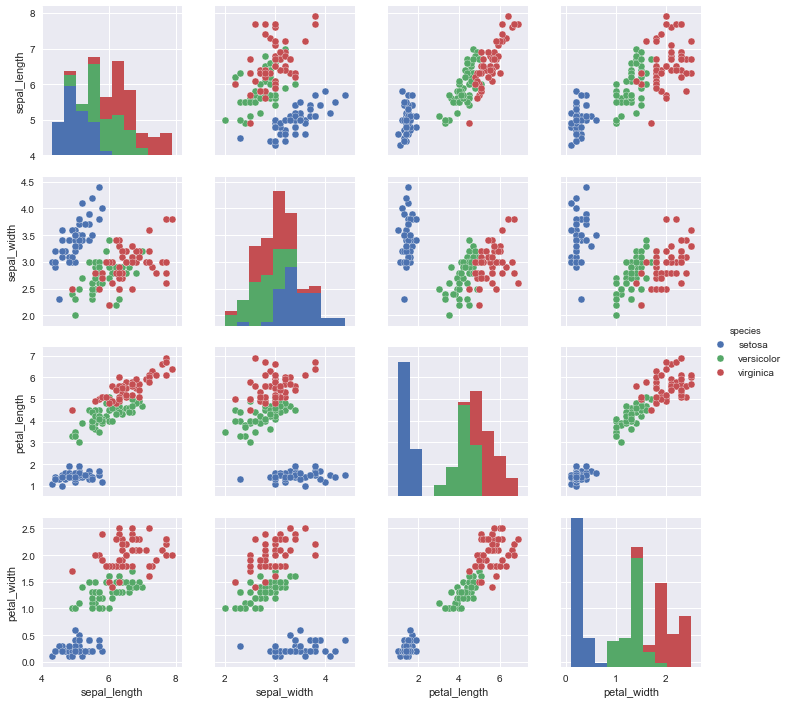### Focus on petal_width vs petal_length¶

In :
iris = iris.rename(index = str, columns = {'sepal_length':'1_sepal_length','sepal_width':'2_sepal_width', 'petal_length':'3_petal_length', 'petal_width':'4_petal_width'})
sns.FacetGrid(iris, hue="species", size=6) .map(plt.scatter,"1_sepal_length", "2_sepal_width", )  .add_legend()
plt.title('Scatter plot')
df1 = iris[["1_sepal_length", "2_sepal_width",'species']]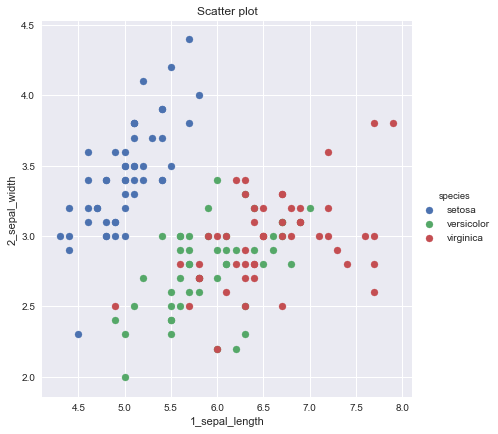# Linear Discriminant Analysis¶

## Visualizing the gaussian estimations and the boundary lines¶

Key assumption - all three Gaussians have the same covariance matrix - hence their shape is the same and only their location differs

In :
#Estimating the parameters
mu_list = np.split(df1.groupby('species').mean().values,[1,2])
sigma = df1.cov().values
pi_list = df1.iloc[:,2].value_counts().values / len(df1)

# Our 2-dimensional distribution will be over variables X and Y
N = 100
X = np.linspace(3, 8, N)
Y = np.linspace(1.5, 5, N)
X, Y = np.meshgrid(X, Y)

#fig = plt.figure(figsize = (10,10))
#ax = fig.gca()
color_list = ['Blues','Greens','Reds']
my_norm = colors.Normalize(vmin=-1.,vmax=1.)

g = sns.FacetGrid(iris, hue="species", size=10, palette = 'colorblind') .map(plt.scatter,"1_sepal_length", "2_sepal_width", )  .add_legend()
my_ax = g.ax

for i,v in enumerate(itertools.combinations([0,1,2],2)):
mu = mu_list[i]
Sigma = sigma

#Computing the cost function for each theta combination
zz = np.array(  [multivariate_gaussian_pdf( np.array([xx,yy]).reshape(-1,1), mu, Sigma)
for xx, yy in zip(np.ravel(X), np.ravel(Y)) ] )

bb = np.array(  [ calculate_boundary(np.array([xx,yy]).reshape(-1,1),mu_list[v].reshape(-1,1),mu_list[v].reshape(-1,1), sigma , .33,.33)
for xx, yy in zip(np.ravel(X), np.ravel(Y)) ] )

#Reshaping the cost values
Z = zz.reshape(X.shape)
B = bb.reshape(X.shape)

#Plot the result in 3D
my_ax.contour( X, Y, Z, 3,cmap = color_list[i] , norm = my_norm, alpha = .3)

my_ax.contour( X, Y, B , levels =  ,cmap = color_list[i]  , norm = my_norm)

# Adjust the limits, ticks and view angle
my_ax.set_xlabel('X')
my_ax.set_ylabel('Y')
my_ax.set_title('LDA: gaussians of each class and boundary lines')

plt.show()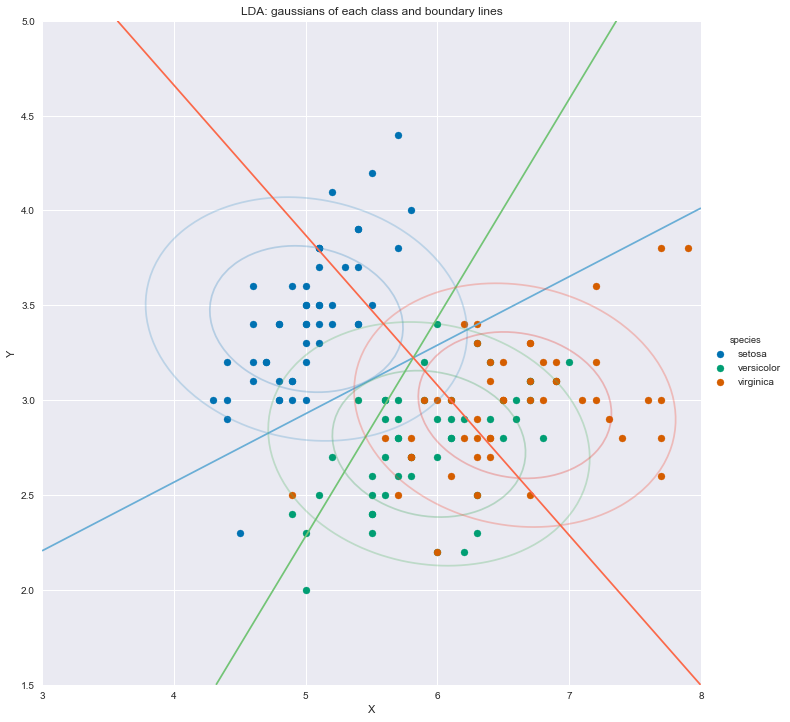## Visualizing the predicted classes based on the LDA score function¶

This is an alternative approach to visualizing the boundary lines. We plot the predicted class value on the entire grid, and then show the boundary lines of the predictions.

In :
# Our 2-dimensional distribution will be over variables X and Y
N = 200
X = np.linspace(3, 8, N)
Y = np.linspace(1.5, 5, N)
X, Y = np.meshgrid(X, Y)

#Initialize seaborn facetplot
g = sns.FacetGrid(iris, hue="species", size=10, palette = 'colorblind') .map(plt.scatter,"1_sepal_length", "2_sepal_width", )  .add_legend()
my_ax = g.ax #Retrieving the faceplot axes

#Computing the predicted class function for each value on the grid
zz = np.array(  [predict_LDA_class( np.array([xx,yy]).reshape(-1,1), mu_list, Sigma, pi_list)
for xx, yy in zip(np.ravel(X), np.ravel(Y)) ] )

#Reshaping the predicted class into the meshgrid shape
Z = zz.reshape(X.shape)

#Plot the filled and boundary contours
my_ax.contourf( X, Y, Z, 2, alpha = .1, colors = ('blue','green','red'))
my_ax.contour( X, Y, Z, 2, alpha = 1, colors = ('blue','green','red'))

my_ax.set_xlabel('X')
my_ax.set_ylabel('Y')
my_ax.set_title('LDA and boundaries')

plt.show()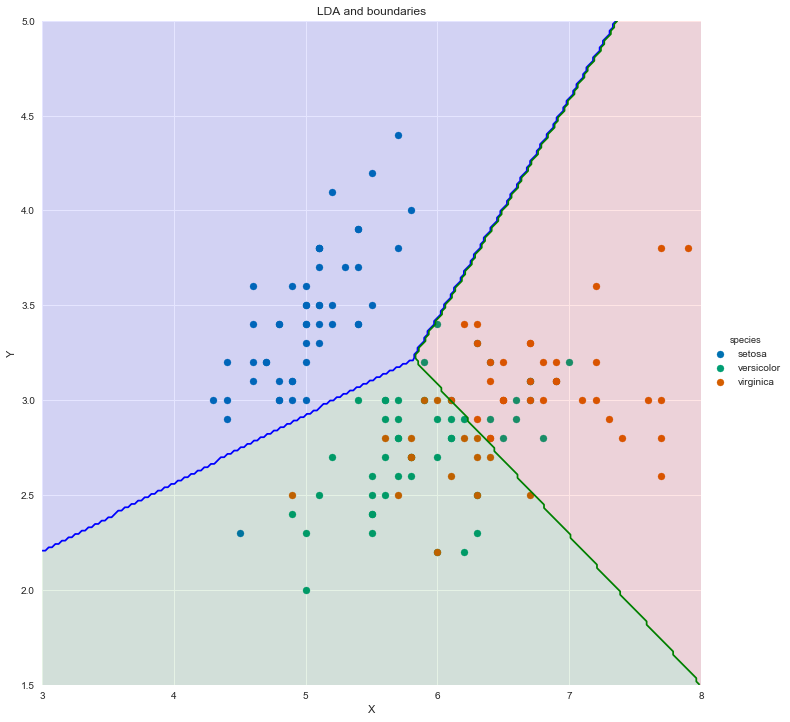### LDA Accuracy¶

In :
#Shape training data
X_data = df1.iloc[:,0:2]
y_labels = df1.iloc[:,2].replace({'setosa':0,'versicolor':1,'virginica':2}).copy()

#Classify and compute accuracy accuracy
y_pred = np.array(  [predict_LDA_class( np.array([xx,yy]).reshape(-1,1), mu_list, Sigma, pi_list)
for xx, yy in zip(np.ravel(X_data.values[:,0]), np.ravel(X_data.values[:,1])) ] )
display(np.mean(y_pred == y_labels))

0.7866666666666666

## Visualizing the Gaussians estimations with different covariance matrices¶

In :
#Estimating the parameters
mu_list = np.split(df1.groupby('species').mean().values,[1,2])
sigma_list = np.split(df1.groupby('species').cov().values,[2,4], axis = 0)
pi_list = df1.iloc[:,2].value_counts().values / len(df1)

# Our 2-dimensional distribution will be over variables X and Y
N = 100
X = np.linspace(3, 8, N)
Y = np.linspace(1.5, 5, N)
X, Y = np.meshgrid(X, Y)

#fig = plt.figure(figsize = (10,10))
#ax = fig.gca()
color_list = ['Blues','Greens','Reds']
my_norm = colors.Normalize(vmin=-1.,vmax=1.)

g = sns.FacetGrid(iris, hue="species", size=10, palette = 'colorblind') .map(plt.scatter, "1_sepal_length", "2_sepal_width",)  .add_legend()
my_ax = g.ax

for i in range(3):
mu = mu_list[i]
Sigma = sigma_list[i]

#Computing the cost function for each theta combination
zz = np.array(  [multivariate_gaussian_pdf( np.array([xx,yy]).reshape(-1,1), mu, Sigma)
for xx, yy in zip(np.ravel(X), np.ravel(Y)) ] )
#Reshaping the cost values
Z = zz.reshape(X.shape)
Zm = np.ma.masked_array(Z, Z < 0.15)

#Plot the result in 3D
my_ax.contour( X, Y, Z, 15, alpha = .3 ,cmap = color_list[i], norm = my_norm)
my_ax.pcolor(X,Y,Zm, alpha = .1, cmap = color_list[i], norm = my_norm)

# Adjust the limits, ticks and view angle
my_ax.set_xlabel('X')
my_ax.set_ylabel('Y')
my_ax.set_title('Multivariate Gaussians with different Sigma ')

plt.show()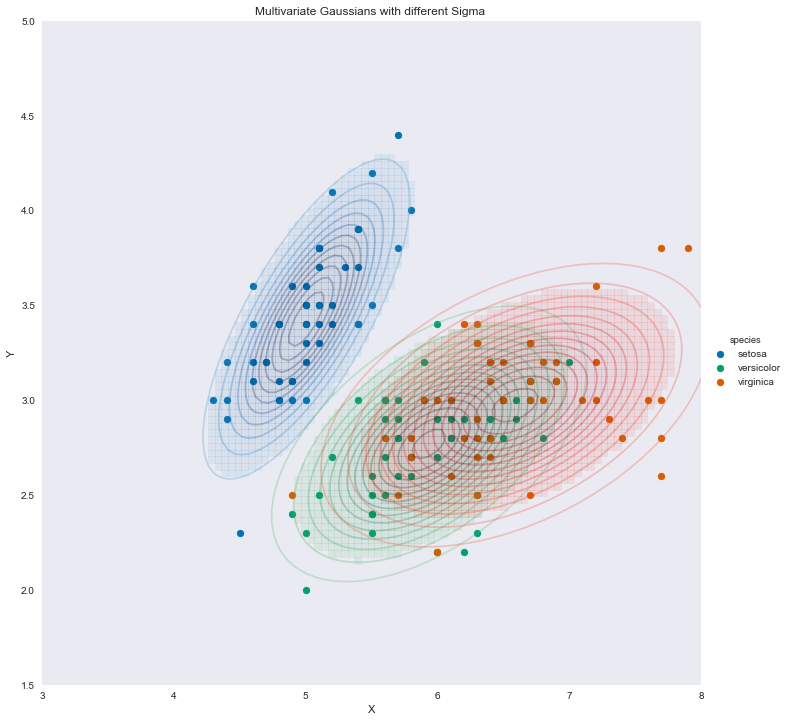## Visualizing the quadratic boundary curves¶

In :
#Estimating the parameters
mu_list = np.split(df1.groupby('species').mean().values,[1,2])
sigma_list = np.split(df1.groupby('species').cov().values,[2,4], axis = 0)
pi_list = df1.iloc[:,2].value_counts().values / len(df1)

# Our 2-dimensional distribution will be over variables X and Y
N = 200
X = np.linspace(4, 8, N)
Y = np.linspace(1.5, 5, N)
X, Y = np.meshgrid(X, Y)

#fig = plt.figure(figsize = (10,10))
#ax = fig.gca()
color_list = ['Blues','Greens','Reds']
my_norm = colors.Normalize(vmin=-1.,vmax=1.)

g = sns.FacetGrid(iris, hue="species", size=10, palette = 'colorblind') .map(plt.scatter, "1_sepal_length", "2_sepal_width",)  .add_legend()
my_ax = g.ax

#Computing the predicted class function for each value on the grid
zz = np.array(  [predict_QDA_class( np.array([xx,yy]).reshape(-1,1), mu_list, sigma_list, pi_list)
for xx, yy in zip(np.ravel(X), np.ravel(Y)) ] )

#Reshaping the predicted class into the meshgrid shape
Z = zz.reshape(X.shape)

#Plot the filled and boundary contours
my_ax.contourf( X, Y, Z, 2, alpha = .1, colors = ('blue','green','red'))
my_ax.contour( X, Y, Z, 2, alpha = 1, colors = ('blue','green','red'))

my_ax.set_xlabel('X')
my_ax.set_ylabel('Y')
my_ax.set_title('QDA and boundaries')

plt.show()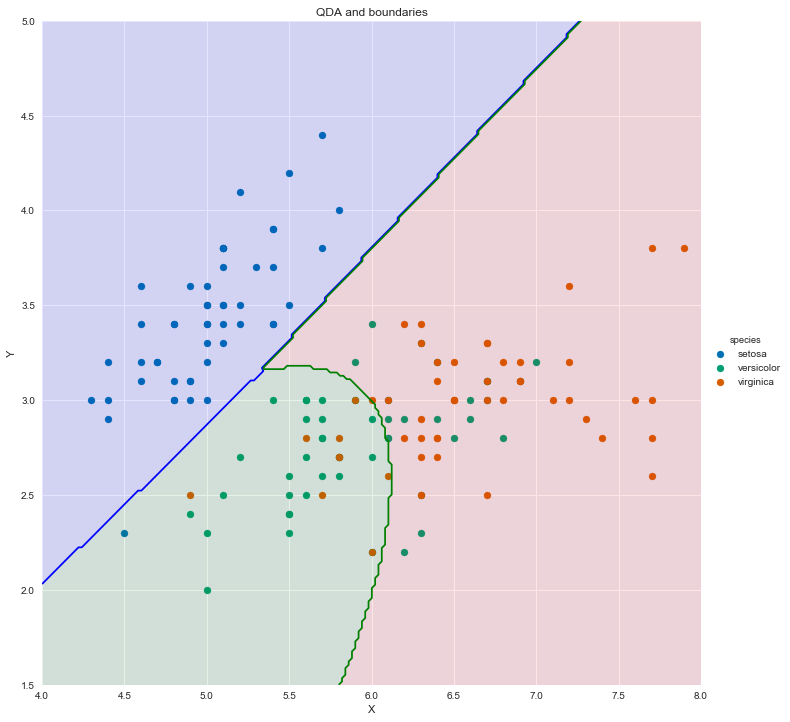# Implementation using Sklearn¶

In :
from sklearn.discriminant_analysis import QuadraticDiscriminantAnalysis
X_data = df1.iloc[:,0:2]
y_labels = df1.iloc[:,2].replace({'setosa':0,'versicolor':1,'virginica':2}).copy()

qda.fit(X_data,y_labels)

Out:
QuadraticDiscriminantAnalysis(priors=None, reg_param=0.0,
store_covariance=True, store_covariances=None, tol=0.0001)
In :
#Estimating the parameters
mu_list = np.split(df1.groupby('species').mean().values,[1,2])
sigma_list = np.split(df1.groupby('species').cov().values,[2,4], axis = 0)
pi_list = df1.iloc[:,2].value_counts().values / len(df1)

# Our 2-dimensional distribution will be over variables X and Y
N = 100
X = np.linspace(4, 8, N)
Y = np.linspace(1.5, 5, N)
X, Y = np.meshgrid(X, Y)

#fig = plt.figure(figsize = (10,10))
#ax = fig.gca()
color_list = ['Blues','Greens','Reds']
my_norm = colors.Normalize(vmin=-1.,vmax=1.)

g = sns.FacetGrid(iris, hue="species", size=10, palette = 'colorblind') .map(plt.scatter, "1_sepal_length", "2_sepal_width",)  .add_legend()
my_ax = g.ax

#Computing the predicted class function for each value on the grid
zz = np.array(  [qda.predict( np.array([[xx,yy]]))
for xx, yy in zip(np.ravel(X), np.ravel(Y)) ] )

#Reshaping the predicted class into the meshgrid shape
Z = zz.reshape(X.shape)

#Plot the filled and boundary contours
my_ax.contourf( X, Y, Z, 2, alpha = .1, colors = ('blue','green','red'))
my_ax.contour( X, Y, Z, 2, alpha = 1, colors = ('blue','green','red'))

my_ax.set_xlabel('X')
my_ax.set_ylabel('Y')
my_ax.set_title('QDA and boundaries')

plt.show()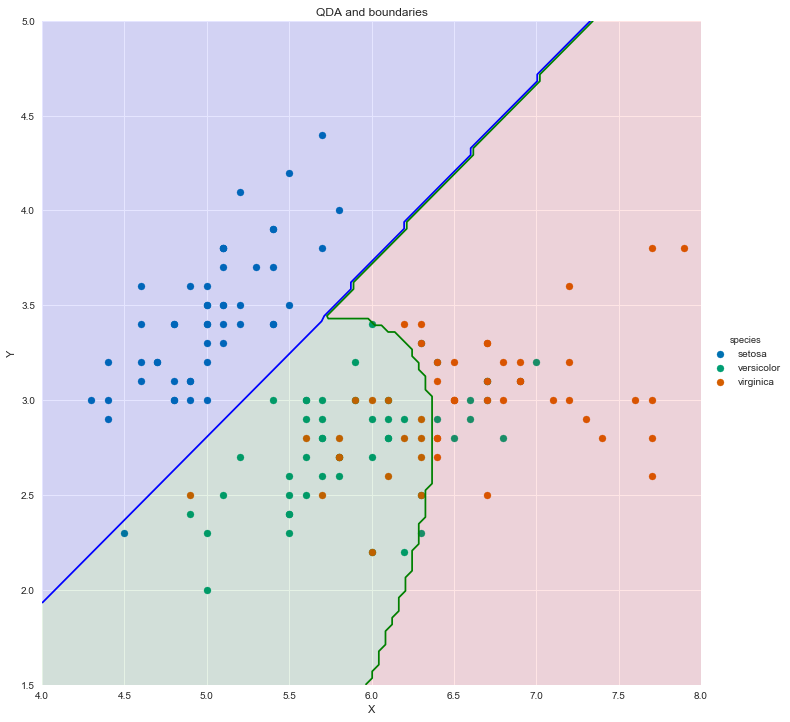### QDA Accuracy¶

In :
#Numpy accuracy
y_pred = np.array(  [predict_QDA_class( np.array([xx,yy]).reshape(-1,1), mu_list, sigma_list, pi_list)
for xx, yy in zip(np.ravel(X_data.values[:,0]), np.ravel(X_data.values[:,1])) ] )
display(np.mean(y_pred == y_labels))

#predict_QDA_class( np.array([xx,yy]).reshape(-1,1), mu_list, sigma_list, pi_list)

#Sklearn accuracy
display(qda.score(X_data,y_labels))

0.7866666666666666
0.8